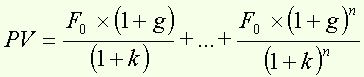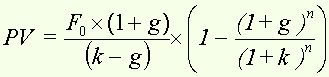Present value of an annuity that grows at rate g for n years

Around the formula...

We assume that F0 x (1+g) is the first year cash flow and grows at rate g over n years.

Then we have :or :assuming that k > g

Thus, a security that pays out 0.8 the first year, growing by 10% for the four following years has, at a discounting rate of 20%, a present value of :

PV = (0,8/0,1) ´ (1 - (1,10/1,20)5) = 2,82